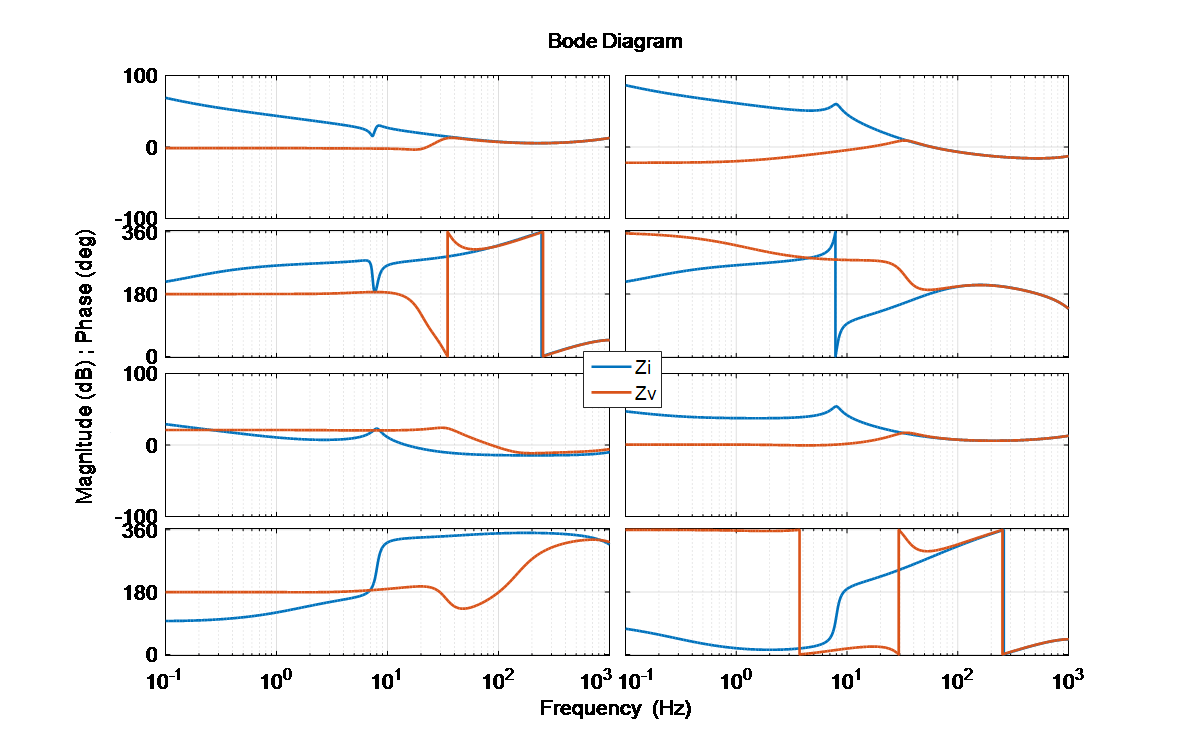LIBRARY

# Analysis of STATCOM Small-Signal Impedance in the Synchronous D-Q FrameFig. 1. STATCOM impedances: with current loop (Zi) and with all loops (Zv).
Small-signal model impedances of grid-tied converters have recently attracted researchers attention and have shown great importance in stability analysis. This paper proposes an impedance model in d-q frame for STATCOMs, including dynamics from synchronization, current, voltage loops and QV droop, and reveals the significant features compared to other types of grid-tied converters: 1) the impedance matrix is strongly coupled in d and q channels due to nearly zero power factor; 2) different behaviors of impedances at low frequency are due to inversed direction of reactive power; and 3) coupled small-signal propagation paths of the voltage are at the point of common coupling from synchronization and ac voltage regulation. All these characteristics render identifying instability patterns difficult using existing knowledge and pinpoint the main contributor to instability among control loops, which is discussed and solved in this paper. To better understand the frequency coupling effects of STATCOMs, the d-q frame impedance model is further transformed in its complex matrix form. An example of possible instability with STATCOMs is presented and analyzed using the proposed model. The impedance model is verified experimentally with a scaled-down STATCOM prototype.
From the model proposed, the following conclusions are extracted. First, because of the inherent operating conditions of a STATCOM, the stability assessment directly from the d-q frame impedances is very difficultas opposed to unity power factor rectifiers and inverters, for which a multivariable stability theorem using the return-ratio matrix product of the upstream and downstream impedances at a given ac interface must be used (for example, the GNC). Second, the ac voltage loop of the STATCOM is found to be the strongest contributor to its terminal impedance and consequently to its small-signal stability conditions as shown in Fig. 1. This loop was shown to effectively mask the PLL dynamics, especially for proper system parameters of transmission grids, previously shown to be critical in grid-tied power converters. Third, and very importantly, the STATCOM impedance is shown to be able to behave both as a CPL rectifier and as a grid-tied inverter injecting power into the grid, from a dynamic standpoint, featuring a negative incremental input-impedance in its Zdd or Zqq impedance elements respectively, as a function of its reactive power injection mode (capacitive or inductive), and as a function of the relative phase between the d-q frame in question and the voltage at the PCC. These are all unique impedance characteristics that make the stability analysis in the presence of STATCOMs more involved than other unity power factor converters. Fourth, alternative control is also modeled, which revealed the impacts on impedance shaping to mitigate the new instability pattern. A 2-STATCOM study case is simulated that validated the above conclusions. Lastly, the model is verified experimentally using an IMU to measure the input-impedance of a 10-kVAR STATCOM prototype, which is shown to match up very well with the impedance obtained using the developed model.